Browse by Topic
Related Topics

# Chemical Solutions: Step-by-Step Chemistry Series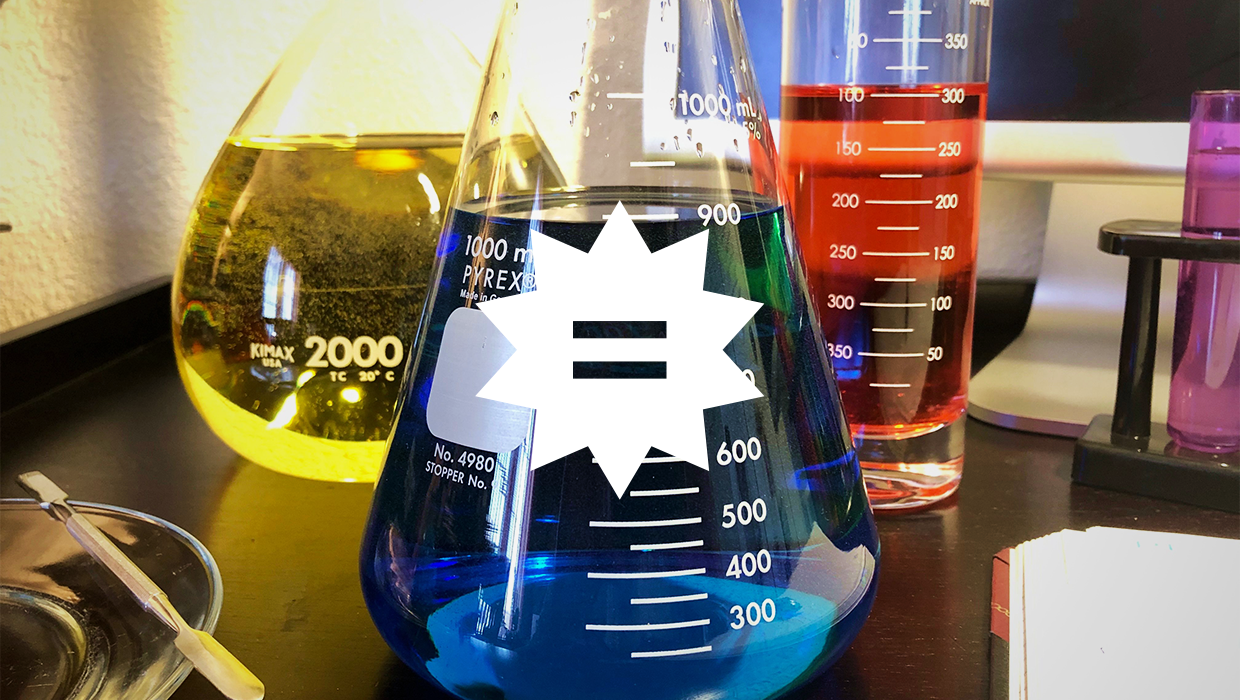Last week, we kicked off a four-part series on Wolfram|Alpha’s step-by-step chemistry offerings with chemical reactions. Future posts will cover chemical structure and bonding along with quantum chemistry. We continue this week with chemical solutions, another foundational component of all chemistry classes.

From the blood in your veins to the oceans covering the planet, solutions are everywhere! Understanding their chemical properties is essential to sustaining life, creating new materials and treating illness. As such, disciplines ranging from biology to material science to the health professions all must be comfortable doing solution-related computations.

To master such calculations, the step-by-step results provide stepwise guides that can be viewed one step at a time or all at once. Read on for example problems covering solute concentration, solution preparation, pKa and colligative properties.

## Concentration

Analysis of chemical solutions begins with determining the concentration of the components in said solution. Step-by-step results are available for computing the amount fraction, mass fraction and molality, in addition to molarity. In all cases, a general framework for solving these concentration problems is provided via the Plan step. Details of which formula to use and how to compute the necessary information are highlighted.

### Example Problem

A 355 mL soft-drink sample contains 0.133 mol of sucrose. What is the molar concentration of sucrose in the sample?

### Step-by-Step Solution

For this problem, enter “molarity of 0.133 mol sucrose in 355 mL water”.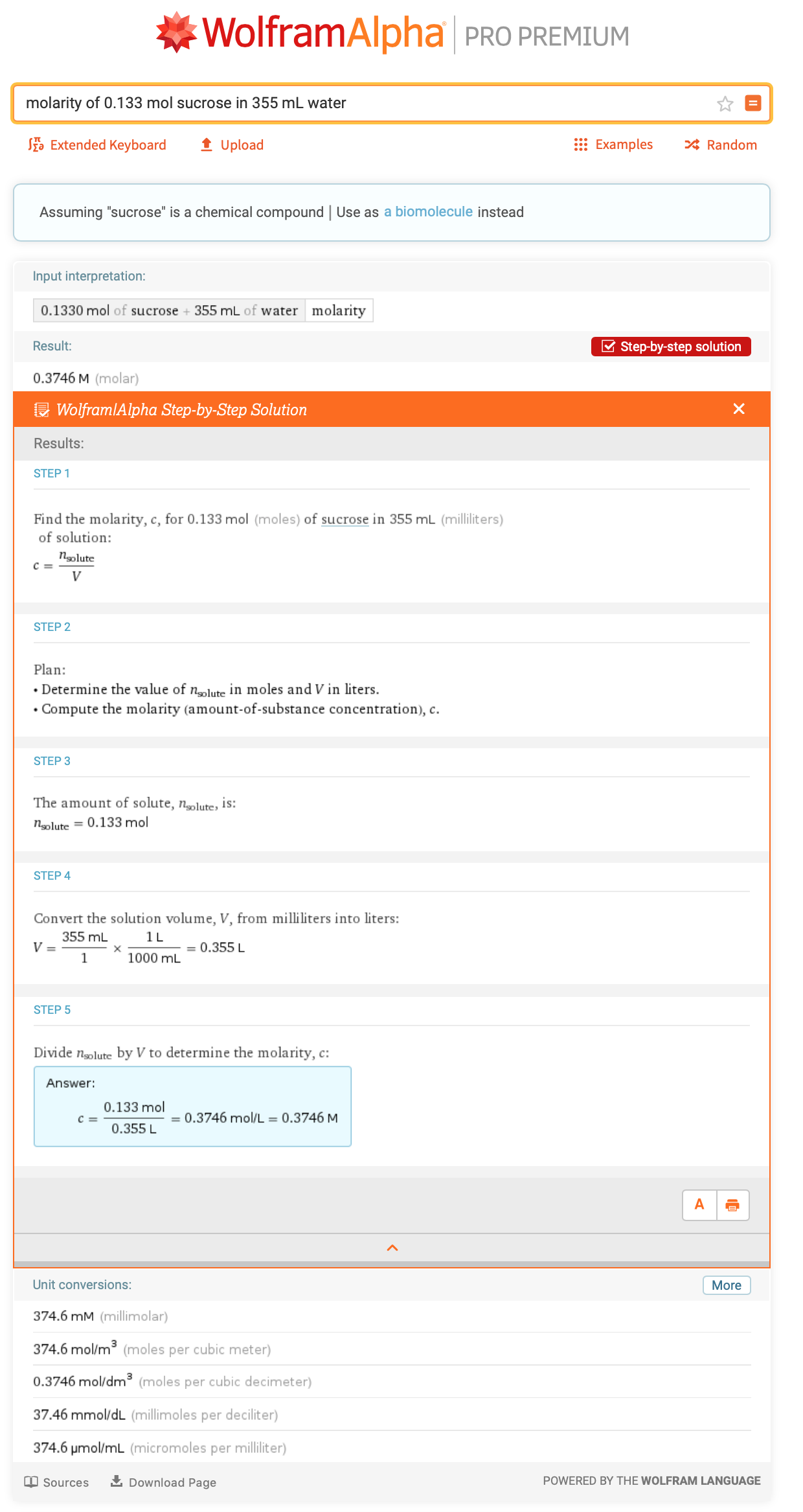## Solution Preparation

Chemical laboratories around the world generate solutions with a desired concentration on a daily basis. Step-by-step results are available for preparing solutions by employing stock solutions of higher concentration and the dilution formula or using the definition of molarity. In both cases, a general framework for solving these types of problems is provided via the Plan step. Details of which formula to use and how to compute the necessary information are highlighted.

### Example Problem

How many milliliters of concentrated HCl are required to make 250 mL of a 2.00 M HCl solution?

### Step-by-Step Solution

To find out how many milliliters you need, enter “prepare 250 mL of 2.00 M HCl from concentrated HCl” since Wolfram|Alpha knows the molarity of concentrated HCl.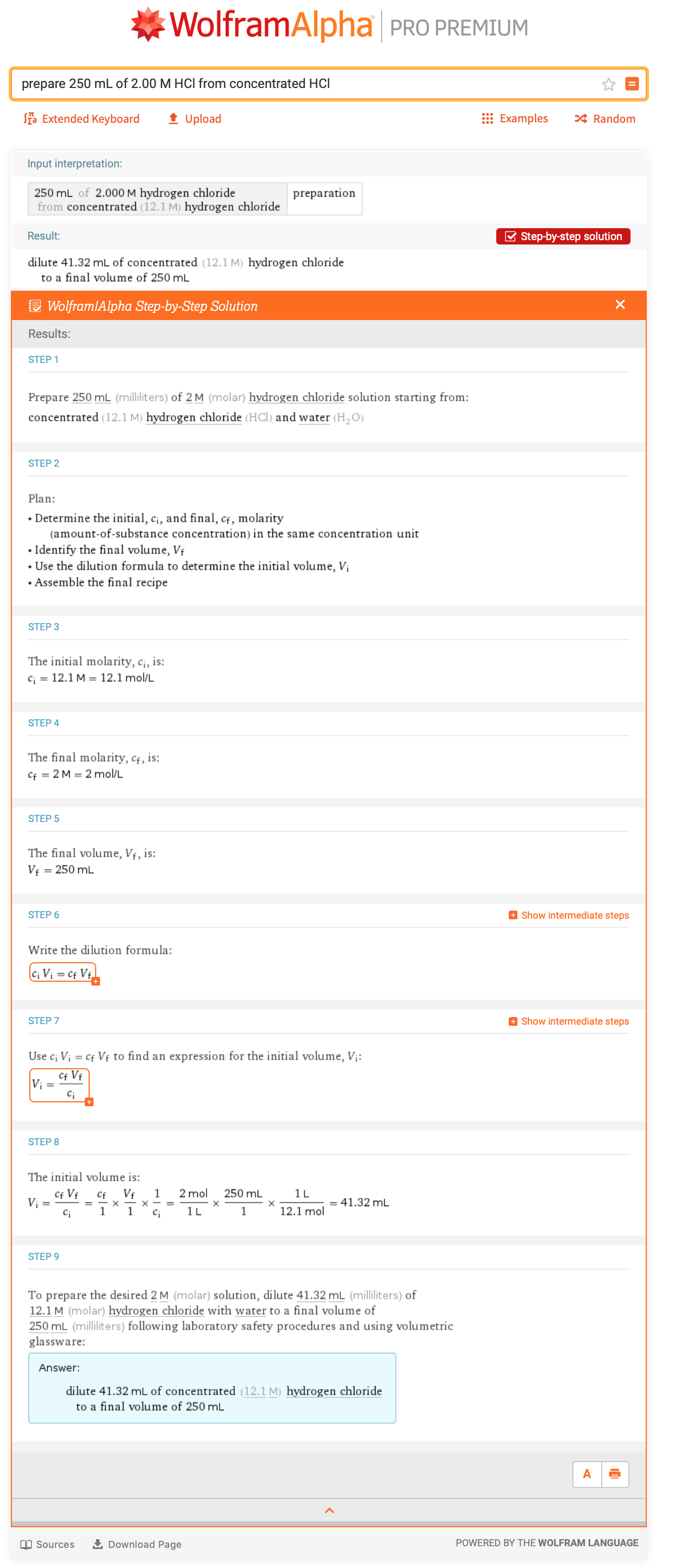## pKa

Understanding how tightly a proton is bound to another atom is central to understanding and predicting chemical reactions involving acids and bases. The experimental value containing this information is the acidity constant, Ka. Acidity constants can span many orders of magnitude, so it is easier to look at the pKa value. The pKa calculator provides easy interconversion of the pKa and the acidity constant.

### Example Problem

Hydrocyanic acid has an acidity constant value of 6.2 × 10–10. What is the corresponding pKa value?

### Step-by-Step Solution

The calculator can be fed known information directly via “pKa Ka=6.2×10^-10”.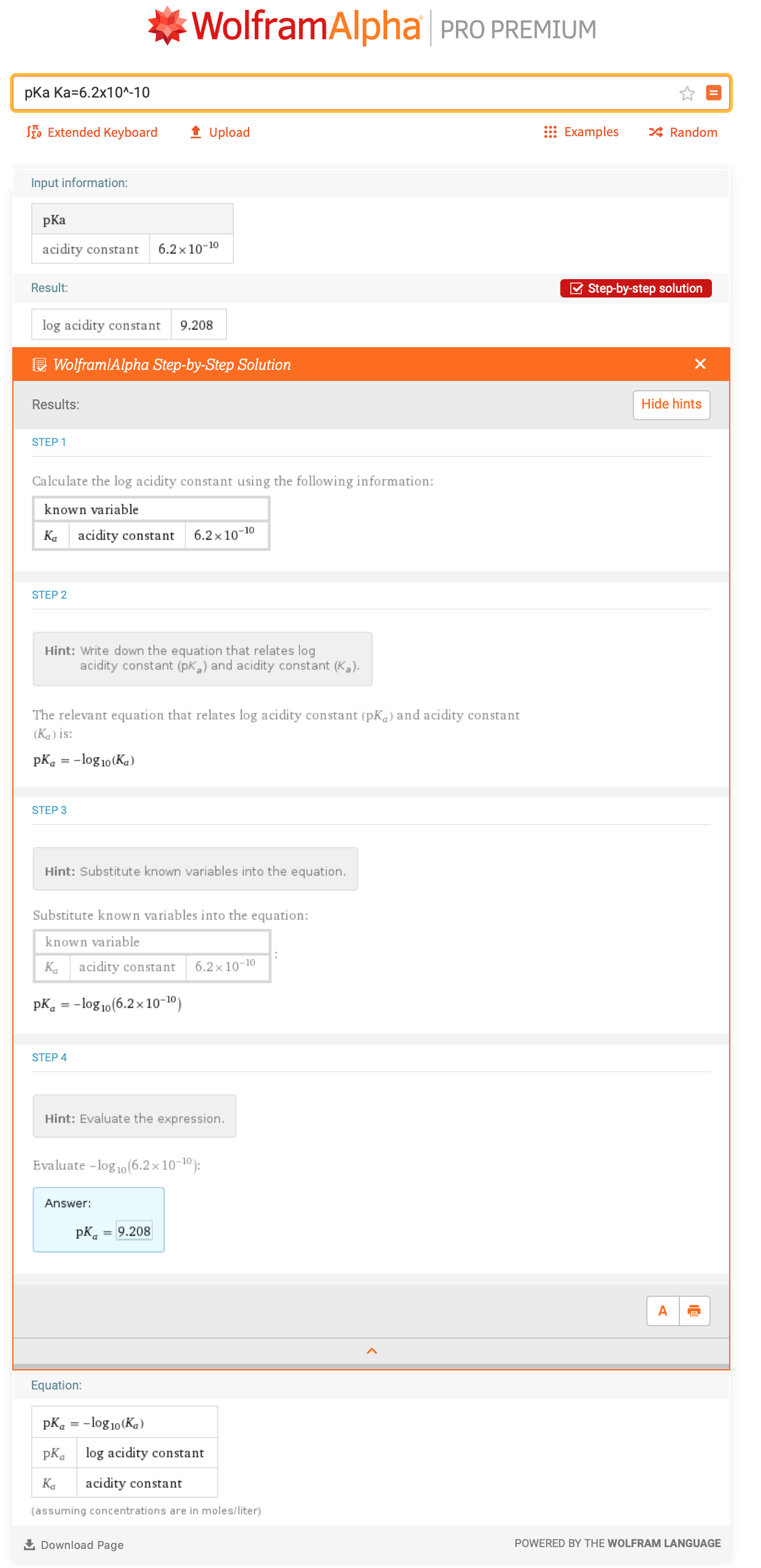## Colligative Properties

The physical properties of a solution differ from the physical properties of the pure solute and solvent composing a solution. Solution properties that primarily depend on the number of solute particles rather than the chemical nature of those particles are known as colligative properties. Step-by-step results are available to compute boiling-point elevations and freezing-point depressions along with the van 't Hoff factor employed in all colligative property formulas.

### Example Problem

What is the boiling point of a 0.33 m solution containing a nonvolatile solute in toluene (Kb = 3.4 K kg/mol), assuming ideal solution behavior?

### Step-by-Step Solution

The necessary calculator can be fed known information directly via “boiling point elevation m=0.33 molal, i=1, Kb=3.4 K kg/mol”.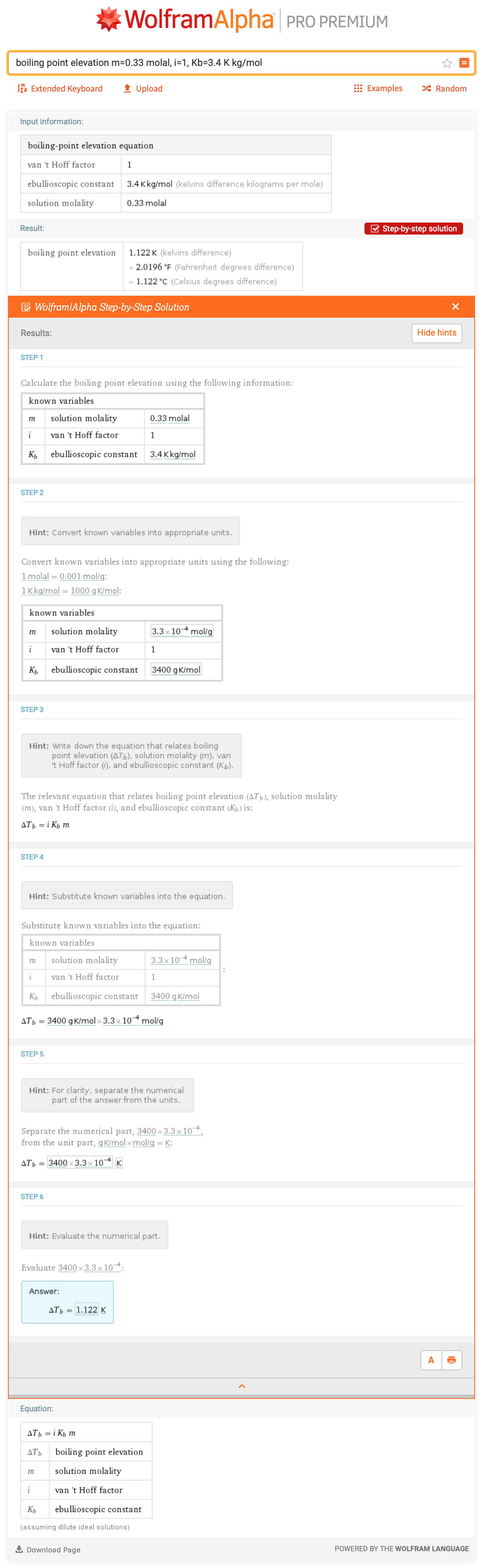## Challenge Problems

Test your chemical solution problem-solving skills by using the Wolfram|Alpha tools described to solve these word problems. Answers will be provided in the next blog post in this series.

1. A good ratio for the salt bath used in old-fashioned ice-cream makers is five cups ice to one cup salt. What is the mass fraction of the resulting mixture?
2. What is the molality of ethylene glycol for a solution that freezes at –5.00 °C?

## Answers to Last Week’s Challenge Problems

Here are the answers to last week’s challenge problems on chemical reactions.

### 1. Compute the molecular mass of acetaminophen. Is the element with the largest atom count also the element with the largest mass percent?

One might be tempted to do two Wolfram|Alpha queries, unless you recall that the atom count and molecular mass are computed during a mass composition calculation.

The molecular mass is 151.165 u. Hydrogen has the largest number of atoms but contributes the smallest total mass. This is a result of the atomic mass of hydrogen being so small.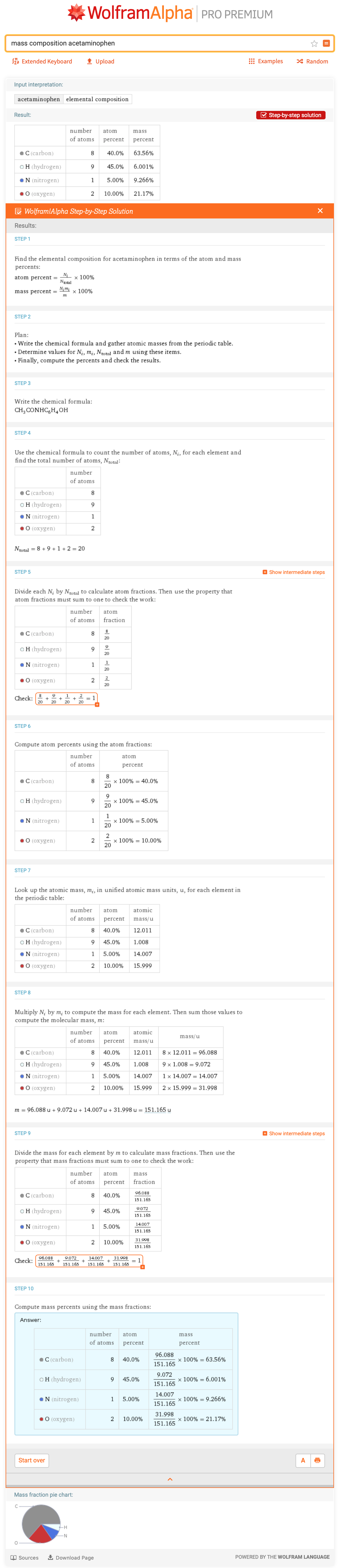### 2. What is the limiting reactant and theoretical yield when 24.8 grams of white phosphorus and 0.200 moles of oxygen react to form 10.0 grams of phosphorus pentoxide?

Similar to the first problem, one might first think it is necessary to submit multiple Wolfram|Alpha queries. But in fact, both answers can be computed at once. Note that Wolfram|Alpha figures out the chemical formulas, does the chemical conversions and balances the chemical equation automatically.

The limiting reagent is oxygen and the theoretical yield of the product is 11.36 grams.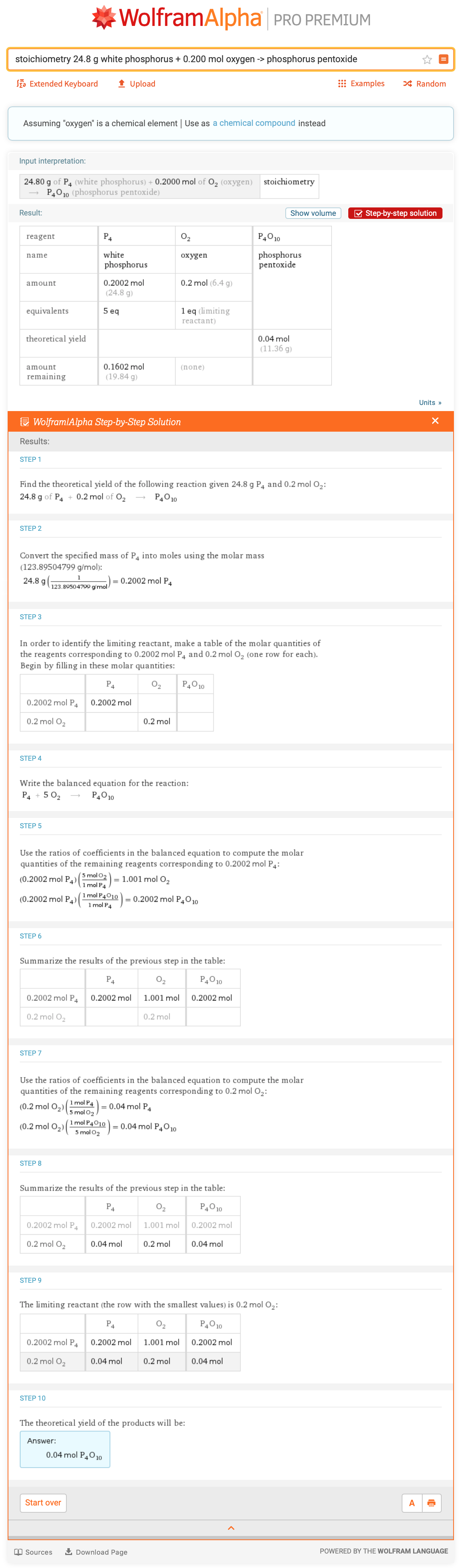See you next week, when we continue with structure and bonding. If you have suggestions for other step-by-step content (in chemistry or other subjects), please let us know! You can reach us by leaving a comment below or sending in feedback at the bottom of any Wolfram|Alpha query page.